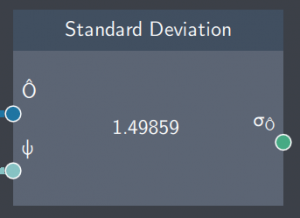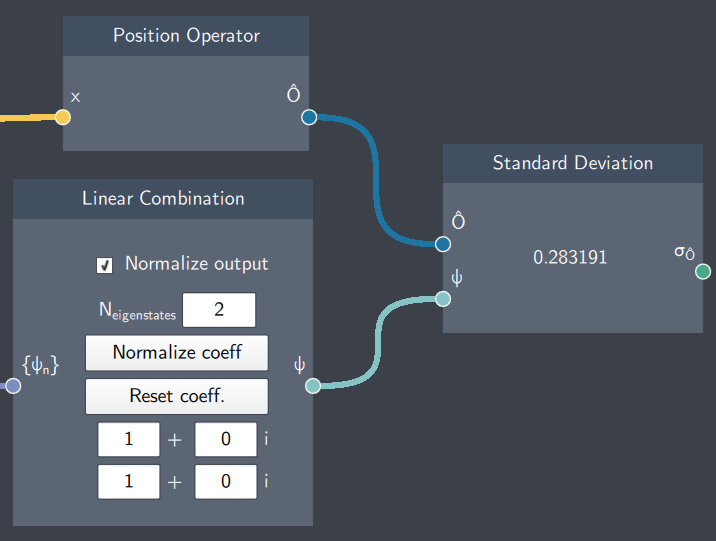# Standard Deviation

## Description

This node calculates the standard deviation of an operator $\hat{O}$ which is defined as $\sigma = \sqrt{\langle\hat{O}^2 \rangle - \langle\hat{O} \rangle ^2}$.## Inputs

The node has the following inputs:

• Operator ($\hat{O}$): Any operator defined in the Operator node such as $\hat{x}$, $\hat{p}$ or $\hat{H}$.
• State ($\psi$): Any state with regard to which the standard deviation needs to be calculated.

## Content

The node displays the standard deviation of the operator.

## Output

• Standard Deviation ($\sigma$): This node outputs the standard deviation of the operator ($\hat{O}$) which is provided in the input.

## Example

In the example below, the standard deviation of the position operator is calculated with regard to a non-stationary state and displayed in the node.